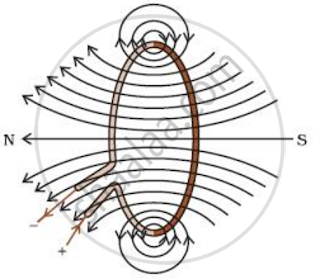# Magnetic Field Due to Current in a Loop (Or Circular Coil)

#### notes

MAGNETIC FIELD DUE TO A CURRENT THROUGH A CIRCULAR LOOP:--Suppose this straight wire is bent in the form of a circular loop and a current is passed through it.

-We know that the magnetic field produced by a current-carrying straight wire depends inversely on the distance from it. Similarly at every point of a current-carrying circular loop, the concentric circles representing the magnetic field around it would become larger and larger as we move away from the wire.

-By the time we reach at the centre of the circular loop, the arcs of these big circles would appear as straight lines. Every point on the wire carrying current would give rise to the magnetic field appearing as straight lines at the center of the loop. By applying the right hand rule, it is easy to check that every section of the wire contributes to the magnetic field lines in the same direction within the loop.

-This rule is also called Maxwell’s corkscrew rule.

Maxwell’s Corkscrew rule: As per Maxwell’s Corkscrew Rule, if the direction of forward movement of screw shows the direction of the current, then the direction of rotation of screw shows the direction of magnetic field.

Clock Face Rule: A current carrying loop works like a disc magnet. The polarity of this magnet can be easily understood with the help of clock face rule. If the current is flowing in anti-clockwise direction, then the face of the loop shows north pole. On the other hand, if the current is flowing in clockwise direction, then the face of the loop shows south pole.

PROPERTIES OF MAGNETIC FIELD:-

• The magnitude; of magnetic field increases with increase in electric current and decreases with decrease in electric current.
• The magnitude of magnetic field; produced by electric current; decreases with increase in distance and vice-versa. The size of concentric circles of magnetic field lines increases with distance from the conductor, which shows that magnetic field decreases with distance.
• Magnetic field lines are always parallel to each other.
• No two field lines cross each other.
If you would like to contribute notes or other learning material, please submit them using the button below.

#### Video Tutorials

We have provided more than 1 series of video tutorials for some topics to help you get a better understanding of the topic.

Series 1

Series 2

### Shaalaa.com

Magnetic Effects of Current part 6 (Circular loop) [00:07:48]
S
0%